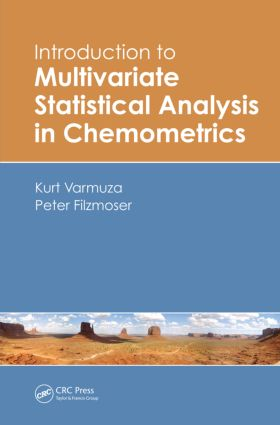# Introduction to Multivariate Statistical Analysis in Chemometrics

## 1st Edition

CRC Press

336 pages | 130 B/W Illus.

##### Purchasing Options:\$ = USD
Hardback: 9781420059472
pub: 2009-02-17
SAVE ~\$34.00
\$170.00
\$136.00
x
eBook (VitalSource) : 9780429145049
pub: 2016-04-19
from \$102.00

FREE Standard Shipping!

### Description

Using formal descriptions, graphical illustrations, practical examples, and R software tools, Introduction to Multivariate Statistical Analysis in Chemometrics presents simple yet thorough explanations of the most important multivariate statistical methods for analyzing chemical data. It includes discussions of various statistical methods, such as principal component analysis, regression analysis, classification methods, and clustering.

Written by a chemometrician and a statistician, the book reflects the practical approach of chemometrics and the more formally oriented one of statistics. To enable a better understanding of the statistical methods, the authors apply them to real data examples from chemistry. They also examine results of the different methods, comparing traditional approaches with their robust counterparts. In addition, the authors use the freely available R package to implement methods, encouraging readers to go through the examples and adapt the procedures to their own problems.

Focusing on the practicality of the methods and the validity of the results, this book offers concise mathematical descriptions of many multivariate methods and employs graphical schemes to visualize key concepts. It effectively imparts a basic understanding of how to apply statistical methods to multivariate scientific data.

### Table of Contents

Introduction

Chemoinformatics–Chemometrics–Statistics

This Book

Historical Remarks about Chemometrics

Bibliography

Starting Examples

Univariate Statistics—A Reminder

Multivariate Data

Definitions

Basic Preprocessing

Covariance and Correlation

Distances and Similarities

Multivariate Outlier Identification

Linear Latent Variables

Summary

Principal Component Analysis (PCA)

Concepts

Number of PCA Components

Centering and Scaling

Outliers and Data Distribution

Robust PCA

Algorithms for PCA

Evaluation and Diagnostics

Complementary Methods for Exploratory Data Analysis

Examples

Summary

Calibration

Concepts

Performance of Regression Models

Ordinary Least Squares Regression

Robust Regression

Variable Selection

Principal Component Regression

Partial Least Squares Regression

Related Methods

Examples

Summary

Classification

Concepts

Linear Classification Methods

Kernel and Prototype Methods

Classification Trees

Artificial Neural Networks

Support Vector Machine

Evaluation

Examples

Summary

Cluster Analysis

Concepts

Distance and Similarity Measures

Partitioning Methods

Hierarchical Clustering Methods

Fuzzy Clustering

Model-Based Clustering

Cluster Validity and Clustering Tendency Measures

Examples

Summary

Preprocessing

Concepts

Smoothing and Differentiation

Multiplicative Signal Correction

Mass Spectral Features

Appendix 1: Symbols and Abbreviations

Appendix 2: Matrix Algebra

Appendix 3: Introduction to R

Index

References appear at the end of each chapter.

### Subject Categories

##### BISAC Subject Codes/Headings:
MAT029000
MATHEMATICS / Probability & Statistics / General
SCI013010
SCIENCE / Chemistry / Analytic
SCI013060
SCIENCE / Chemistry / Industrial & Technical